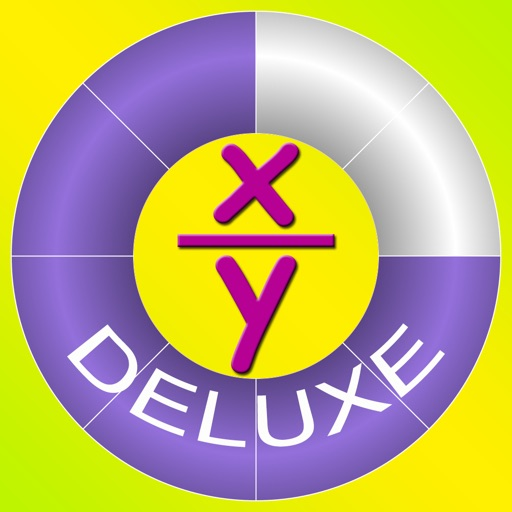## Learn and practice the fundamental concepts about fractions including addition, subtraction, multiplication and division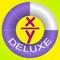# Basic Fraction Deluxe

by Explorer Technologies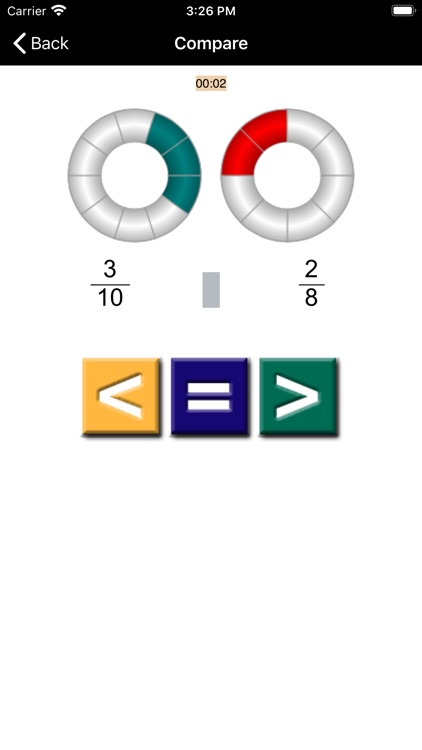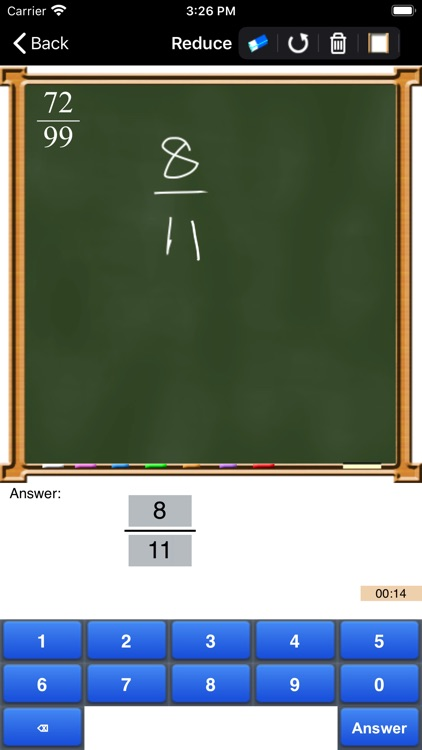Learn and practice the fundamental concepts about fractions including addition, subtraction, multiplication and division. Extensions to fraction include algebra (introduction through Find x questions), decimal and percentage.### App Details

Version
1.6
Rating
NA
Size
12Mb
Genre
Education
Last updated
December 21, 2020
Release date
October 28, 2011

### App Screenshots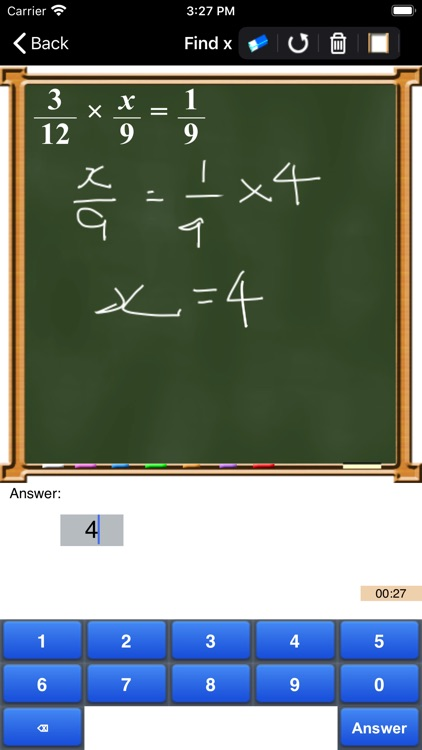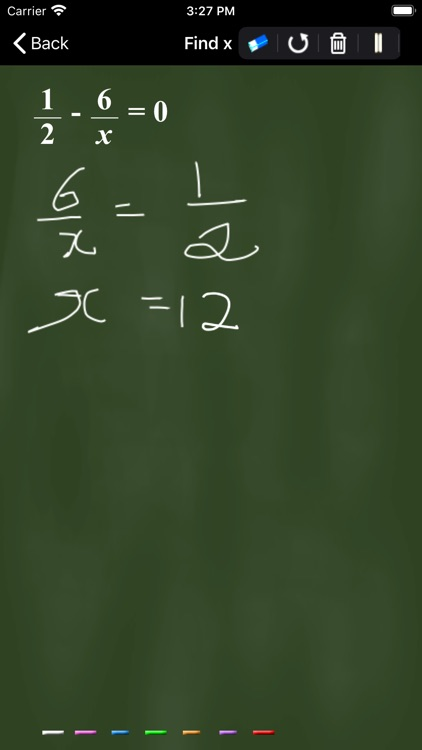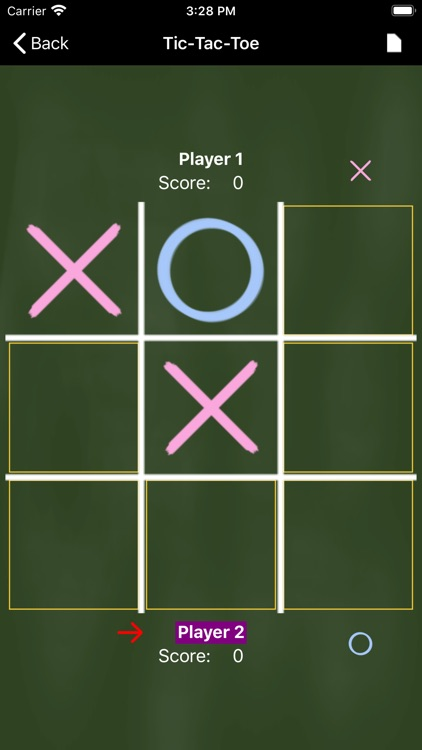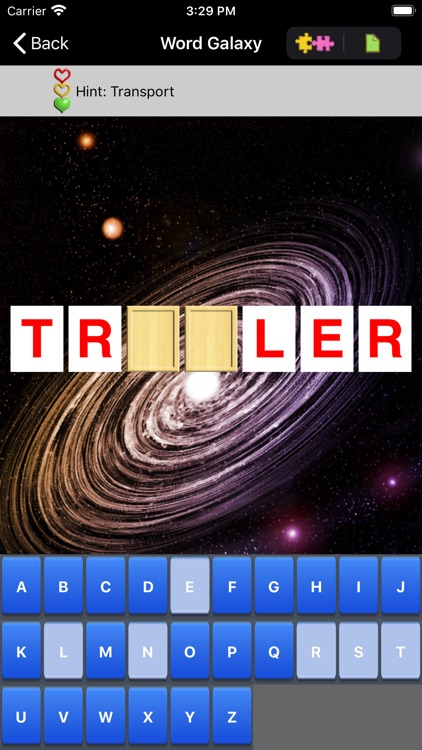### App Store Description

Learn and practice the fundamental concepts about fractions including addition, subtraction, multiplication and division. Extensions to fraction include algebra (introduction through Find x questions), decimal and percentage.

2 games are provided to make these fraction drills fun. Tic-Tac-Toe is a 2-player game where players take turn to mark the board and work on fractions. Word Galaxy is a single-player game that will flex the left and right hemisphere of the brain while the player solves fraction questions and a word/phrase puzzle.

Suitable for kids 12 years old and below.

Features:
- Identify fractions with visual images
- Compare 2 fractions with visual aid
- Reduce/Simplify fraction
- Perform addition,subtraction,multiplication, or division on 2 fractions
- Visual feedback is provided for both correct and wrong answers.
- help pages
- full rotational support
- doodling for rough work (please see screen shots for examples)
- Find X feature: solve algebraic fraction equation
- Video links to web casts on Fraction
- Convert fraction to decimal and percentage
- Single-player word/phrase puzzle game
- Decimal and Percentage
- User defined random function
- Enhanced Help page

Settings page to configure:
- Time delay before showing the next question
- Level of difficulty by setting the max denominator
- Answers in mixed fraction or improper fraction
- Show solution to arithmetic and simplify/reduce type of questions
- Turn timer on/off